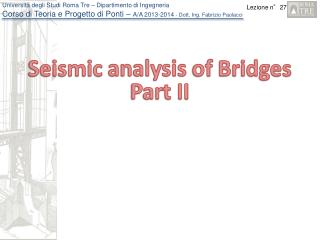Download PresentationSeismic analysis of Bridges Part II

Seismic analysis of Bridges Part II - PowerPoint PPT Presentation

Seismic analysis of Bridges Part II. Analysis methods Simplified non-linear analysis: Simply supported Bridges. Most of the bridges are realized with simply supported deck.I am the owner, or an agent authorized to act on behalf of the owner, of the copyrighted work described.
Download PresentationSeismic analysis of Bridges Part II

Download Policy: Content on the Website is provided to you AS IS for your information and personal use and may not be sold / licensed / shared on other websites without getting consent from its author.While downloading, if for some reason you are not able to download a presentation, the publisher may have deleted the file from their server.

- - - - - - - - - - - - - - - - - - - - - - - - - - E N D - - - - - - - - - - - - - - - - - - - - - - - - - -
Presentation Transcript
1. Seismicanalysis of BridgesPart II

2. Analysis methodsSimplified non-linear analysis: Simply supported Bridges Most of the bridges are realized with simply supported deck. For the evaluation of the seismic behaviour of as-built condition of the retrofitted condition that maintain the static scheme (presence of discontinuity of the deck) a simplified methodology can be adopted. It is base on the following steps: The pier can be considered a simple oscillator with a mass given by the tributary mass relative to the deck and the mass of the pier. The non linearity can be considered as concentrated at the pier base and assumed of elasto-perfectly plastic type The capacity of the pier represented by the shear displacement curve evaluated starting to the yielding and ultimate rotation on the plastic hinge at the pier base The response is evaluated by Push-over analysis using the N2 method The verification is made in term of ductile and fragile mechanisms

3. Analysis methodsSimplified non-linear analysis: Simply supported Bridges Transversal direction: determinationof the SDOF characteristics Mass of the simplified system Effective height the pier

4. Analysis methodsSimplified non-linear analysis: Simply supported Bridges Transversal direction: determinationof the capacity Length of the plastic hinge On the basis of the simplified model the capacity of the pier can be evaluated starting form the linearized Moment-Curvature relationship and evaluating the yielding and ultimate top displacements using the following formula

5. Analysis methodsSimplified non-linear analysis: Simply supported Bridges Transversal direction: Evaluationof the performance pointby N2 Principle of equal displacement Principle of equal Energy

6. Analysis methodsSimplified non-linear analysis: Simply supported Bridges Evaluation of strength reduction factors for earthquake-resistant design, Miranda Bertero ( 1994) – E. Spectra vol 10 n° 2 (behaviour factor)

7. Analysis methodsSimplified non-linear analysis: Simply supported Bridges As soon as we have found the global multilinear capacity curve of the bridge, built considering all the piers in parallel, the above procedure can be easily applied considering the global mass as the sum of the single masses

8. Analysis methodsSimplified non-linear analysis: Continuous deck bridges Mono-modal method Bi-linearization Distribution of force Participation factor V * u*

9. Analysis methodsSimplified non-linear analysis: Continuous deck bridges Evaluation of the maximum displacement V * u* To be compared With the capacity

10. Example

11. Simply supported bridge (ordinary bridge) Geometry 5 3 Data 2 4 1 Class IV Soil B VS,30 > 500m/s Concrete fc = 25 MPa Steel fsy = 400 MPa

12. Simply supported bridge Definition of the seismic input Life safety level (SLV) PR=10%  TR=949 y. Nominal life (ordinary bridge): VN= 50 years Class: IV  Cu = 2 Reference life : VR = Cu  VN = 100 years Soil B Collapse prevention (SLC) PR=5%  TR=1950 y. NTC08 provide discrete TR. Therefore an interpolation of data is needed

13. Simply supported bridge Definition of the seismic input Life safety level (SLV) PR=10%  TR=949 y. Nominal life (ordinary bridge): VN= 50 years Class: IV  Cu = 2 Reference life : VR = Cu  VN = 100 years Soil B Collapse prevention (SLC) PR=5%  TR=1950 y. NTC08 provide discrete TR. Therefore an interpolation of data is needed

14. Simply supported bridge Evaluation of weight and mass (pier 5) Mass of the deck Height of the deck respect to ground Total weight of the pier cap Height of the pier cap Linear weight of the pier Height of the pier Total weight of the pier Height of foundation Axial force on the pier Total mass 10.0 m

15. Simply supported bridge Evaluation of Moment-rotation of the pier base Concrete: Kent-Park model Steel N=5437 kN Bi-linearization

16. Simply supported bridge Evaluation of the capacity of the piers (Force-displacement law) Pier 5

17. Simply supported bridge Evaluation of the seismic Demand (transversal direction) Fundamental period (T > Tc) equal displacement Spectral acceleration (SLV) Spectral Displacement Base-shear Base Moment

18. Simply supported bridge Evaluation of the seismic Capacity (Longitudinal direction) Bilinearization of the Multilinear law

19. Simply supported bridge Evaluation of the seismic Demand (Longitudinal direction) (non-linear response) For example for the pier 5

20. Simply supported bridge Verification format (Ductile mechanisms) Pier 5

21. Simply supported bridge Verification format (Ductile mechanisms)

22. Simply supported bridge Verification format (Fragile mechanisms) Axial force contribution Concrete contribution Reinforcement contribution

23. Simply supported bridge Verification format (Fragile mechanisms)

24. Simply supported bridge Verification format (Fragile mechanisms) Pier 5

25. Simply supported bridge SLV SLC The level of safety is encountered both for SLV and SLC In conclusion the bridge does not have an enough level of safety both with respect to ductile mechanism and to fragile one A retrofitting action has to be adopted.